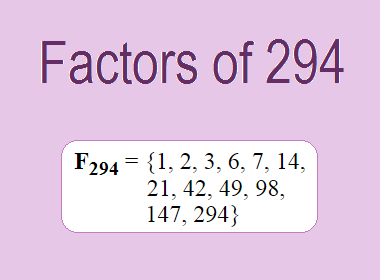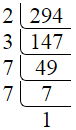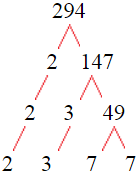# Factors of 294The factors of 294 are 1, 2, 3, 6, 7, 14, 21, 42, 49, 98, 147, and 294 i.e. F294 = {1, 2, 3, 6, 7, 14, 21, 42, 49, 98, 147, 294}. The factors of 294 are all the numbers that can divide 294 without leaving a remainder.

We can check if these numbers are factors of 294 by dividing 294 by each of them. If the result is a whole number, then the number is a factor of 294. Let's do this for each of the numbers listed above:

·        1 is a factor of 294 because 294 divided by 1 is 294.

·        2 is a factor of 294 because 294 divided by 2 is 147.

·        3 is a factor of 294 because 294 divided by 3 is 98.

·        6 is a factor of 294 because 294 divided by 6 is 49.

·        7 is a factor of 294 because 294 divided by 7 is 42.

·        14 is a factor of 294 because 294 divided by 14 is 21.

·        21 is a factor of 294 because 294 divided by 21 is 14.

·        42 is a factor of 294 because 294 divided by 42 is 7.

·        49 is a factor of 294 because 294 divided by 49 is 6.

·        98 is a factor of 294 because 294 divided by 98 is 3.

·        147 is a factor of 294 because 294 divided by 147 is 2.

·        294 is a factor of 294 because 294 divided by 294 is 1.

## How to Find Factors of 294?

1 and the number itself are the factors of every number. So, 1 and 294 are two factors of 294. To find the other factors of 294, we can start by dividing 294 by the numbers between 1 and 294. If we divide 294 by 2, we get a remainder of 0. Therefore, 2 is a factor of 294. If we divide 294 by 3, we get a remainder of 0. Therefore, 3 is a factor of 294.

Next, we can check if 4 is a factor of 294. If we divide 294 by 4, we get a remainder of 2. Therefore, 4 is not a factor of 294. We can continue this process for all the possible factors of 294.

Through this process, we can find that the factors of 294 are 1, 2, 3, 6, 7, 14, 21, 42, 49, 98, 147, and 294. These are the only numbers that can divide 294 without leaving a remainder.

********************

********************

## Properties of the Factors of 294

The factors of 294 have some interesting properties. One of the properties is that the sum of the factors of 294 is equal to 684. We can see this by adding all the factors of 294 together:

1 + 2 + 3 + 6 + 7 + 14 + 21 + 42 + 49 + 98 + 147 + 294 = 684

Another property of the factors of 294 is that the prime factors of 294 are 2, 3, and 7 only.

## Applications of the Factors of 294

The factors of 294 have several applications in mathematics. One of the applications is in finding the highest common factor (HCF) of two or more numbers. The HCF is the largest factor that two or more numbers have in common. For example, to find the HCF of 294 and 147, we need to find the factors of both numbers and identify the largest factor they have in common. The factors of 294 are 1, 2, 3, 6, 7, 14, 21, 42, 49, 98, 147, and 294. The factors of 147 are 1, 3, 7, 21, 49, and 147. The largest factor that they have in common is 147. Therefore, the HCF of 294 and 147 is 147.

Another application of the factors of 294 is in prime factorization. Prime factorization is the process of expressing a number as the product of its prime factors. The prime factors of 294 are 2, 3, and 7 since these are the only prime numbers that can divide 294 without leaving a remainder. Therefore, we can express 294 as:

294 = 2 × 3 × 7 × 7

We can do prime factorization by division and factor tree method also. Here is the prime factorization of 294 by division method,294 = 2 × 3 × 7 × 7

Here is the prime factorization of 294 by the factor tree method,294 = 2 × 3 × 7 × 7

## Conclusion

The factors of 294 are the numbers that can divide 294 without leaving a remainder. The factors of 294 are 1, 2, 3, 6, 7, 14, 21, 42, 49, 98, 147, and 294. The factors of 294 have some interesting properties, such as having a sum of 684. The factors of 294 have several applications in mathematics, such as finding the highest common factor and prime factorization.